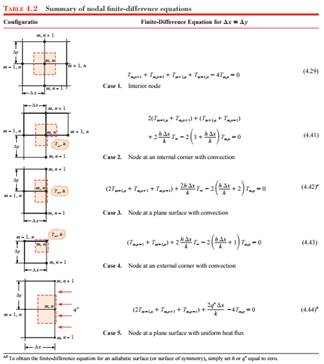### Create an Account

Already have account?

### Forgot Your Password ?

Home / Questions / Consider nodal configuration 3 of Table 42 Derive the finite-difference equations under st...

# Consider nodal configuration 3 of Table 42 Derive the finite-difference equations under steady state conditions for the following situations a The boundary is insulated Explain how Equation

Consider nodal configuration 3 of Table 4.2. Derive the finite-difference equations under steady-state conditions for the following situations.

(a) The boundary is insulated. Explain how Equation 4.42 can be modified to agree with your result.

(b) The boundary is subjected to a constant heat fluxJun 27 2020 View more View Less

#### Answer (Solved)Subscribe To Get Solution